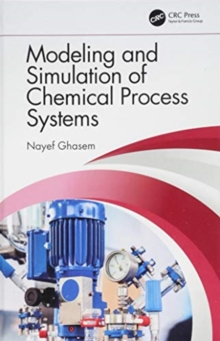Supporting your high street Find out how »
• My Account# Modeling and Simulation of Chemical Process Systems Hardback

#### Description

In this textbook, the author teaches readers how to model and simulate a unit process operation through developing mathematical model equations, solving model equations manually, and comparing results with those simulated through software.

It covers both lumped parameter systems and distributed parameter systems, as well as using MATLAB and Simulink to solve the system model equations for both.

Simplified partial differential equations are solved using COMSOL, an effective tool to solve PDE, using the fine element method.

This book includes end of chapter problems and worked examples, and summarizes reader goals at the beginning of each chapter.

#### Information

• Format: Hardback
• Pages: 502 pages, 18 Tables, black and white; 386 Illustrations, black and white
• Publisher: Taylor & Francis Ltd
• Publication Date:
• Category: Mathematical modelling
• ISBN: 9781138568518

£115.00

£106.15

on all orders

###### Pick up orders

from local bookshops